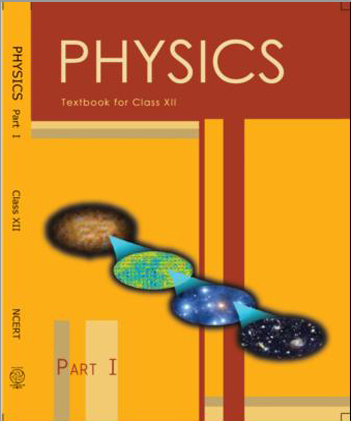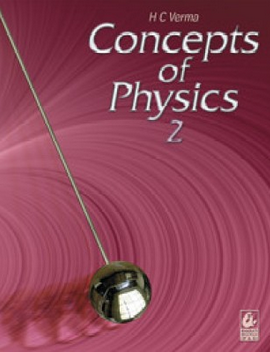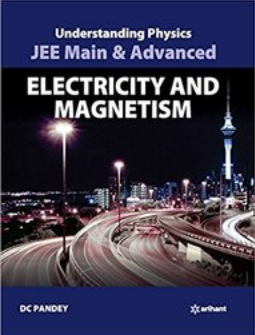# Electrostatics   Share

## What is Electrostatics

In your childhood, have you played the plastic chair and towel game? If you haven’t played yet, then let's try this interesting game. For this game, you require 2-3 people, one plastic chair and a towel. First of all, one person should sit on the chair with both feet above the ground (no body part should in contact with the ground or any other thing except chair) and then one person take a towel and hits the back of the chair for 30 seconds. When someone will touch the person who is sitting on the chair, he or she will get a very mild electric shock. Now if you are wondering how can this happen, then the concepts of electrostatics will help you to understand this phenomenon. Electrostatics constitutes of two words “Electro” means electron or charge and “Static” means at rest. So in this plastic chair and towel game, due to the beating of the towel, the charges are generated and we get shocked due to these static charges. The similar phenomenon is when we comb our hair on a dry day and bring the comb close to tiny pieces of paper, we note that they are swiftly attracted by the comb.## Electrostatics- Topics

Electric charge, Conductor and Insulators, Charging of a body, Coulomb's law, Electric Field, Electric Potential Energy, Electric Potential, Relation between Electric Field and Potential, Equipotential Surfaces, Electric dipole, Gauss’s law, Properties of a conductor, Electric Field and Potential due to Charged Spherical Shell or Solid Conducting Surface, Capacitance, Energy Stored in a charged Capacitor, Capacitors in series and parallel, Two laws in Capacitance, Energy Density, C-R Circuits

## Overview of Electrostatics

In electrostatics, we are only concerned about the charge which is at rest, meaning the charge is not moving like the electric current. In this chapter, you will find a lot of similarities between electrostatics and gravitation. If you have weak concepts in gravitation then read that chapter first. Just like gravitation, we have charge (electrons) in place of mass and the relation of Electric force is also similar, here Electric Force exerted by one point charge on another act along the line between charges. It varies inversely as the square of the distance separating the charges and is proportional to the product of charges. The basic difference between gravitational force and electric force is that the former one only attracts while the latter one attracts only if the charges are of opposite nature (positive and negative) and a repulsive force acts if the charges have same nature.  Electrons are known to have a negative charge while protons have a positive charge. When you will further proceed you will find other similar concepts to Gravitation like Electric Field, and Electric potential. And then you will get to know about Gauss’s Law which helps in easy calculation of Electric Field and it is a very important concept because calculating electric field by using integration can be a mess sometimes. Another important concept in this chapter is an electric dipole (forms when a pair of equal and opposite point charges are separated by a fixed distance) which occurs in nature in a variety of situations. The Hydrogen Fluoride molecule (HF) is a typical example of an electric dipole. Every electric dipole is characterized by its electric dipole moment which is a vector “p” directed from negative to the positive charge. In gravitation, we do not have dipoles can you suggest why?

Prepare Online for JEE Main/NEET

Crack JEE 2021 with JEE/NEET Online Preparation Program

Yes, you are right, the gravitational force is always attractive hence no dipole formation.

Further, in this chapter, you will learn about another important concept of capacitors. The capacitor is a device which can store electric charge and potential energy. Capacitors have various applications in real-life like the flashlights in cameras, in fan motors etc.

## Formulas for Electrostatics

• Electric Force,where q1 and q2 are point charges

• Electric Field,• Electric Potential Energy,• Electric Potential,• Dipole moment,where 2a is the distance between the two charges

• Capacitance,• Equivalent Capacitance

• Parallel arrangement,• Series arrangement,## How to prepare Electrostatics?

Before solving questions of electrostatics first you should be comfortable with the concepts which you have read in gravitation, because of the similarity it is very important for you to have good hold in gravitational concepts. In this chapter, most of the problems will ask you to calculate the electric field or electric potential, so practice more on these concepts. Also in some problems, you might find the use of mechanics concepts, so keep revising the mechanics part as well. This chapter is more of a concept based rather than memory based, so practice is the key here.

## Tips for Electrostatics

• Don’t just memorize formulas of electric field and potential of different objects, first prove them by yourself with the help of derivations and then memorize.

• Keep in mind the units and dimensional formula of various entities, because sometimes questions are directly asked to convert one entity to another. Example - If Electric Force is given then what is the electric field at a point P.

• Use of vectors is very necessary, sometimes questions are directly asked about the direction of Electric Field or Force, etc.

• Don’t get confused over parallel and series combination of capacitors, always remember the formula for calculating equivalent capacitance in both these arrangements.

• Give regular online topic-wise mock tests to build conceptual knowledge.

• Before solving a question, first of all, look at what quantities are given and then think of a strategy to solve the question.

## Books For Electrostatics

First of all, read the NCERT book, each and every concept is explained in a very simple language and examples given in the book are also good. After reading you could solve questions from NCERT Exemplar. And if you are preparing for competitive exams like JEE or NEET you could follow Understanding Physics by DC Pandey.

## Physics Chapter wise Notes For Engineering and Medical Exams

 Chapters No. Chapters Name Chapter 1 Physics and Measurement Chapter 2 Kinematics Chapter 3 Laws of motion Chapter 4 Work Energy and Power Chapter 5 Rotational Motion Chapter 6 Gravitation Chapter 7 Properties of Solids and Liquids Chapter 8 Kinetic theory of Gases Chapter 9 Thermodynamics Chapter 10 Oscillations and Waves Chapter 12 Current Electricity Chapter 13 Magnetic Effects of Current and Magnetism Chapter 14 Electromagnetic Induction and Alternating currents Chapter 15 Electromagnetic Waves Chapter 16 Optics Chapter 17 Dual Nature of Matter and Radiation Chapter  18 Atoms And Nuclei Chapter 19 Electronic devices Chapter 20 Communication Systems Chapter 21 Experimental skills

### Topics from Electrostatics

• Electric charges ( JEE Main ) (8 concepts)
• Basic Properties of Electric Charge ( JEE Main ) (4 concepts)
• Electrostatics of Conductors ( JEE Main ) (3 concepts)
• Charge on surface ( JEE Main ) (2 concepts)
• Continuous Charge Distribution ( JEE Main ) (4 concepts)
• Methods of charging,Charging by Induction ( JEE Main ) (3 concepts)
• Coulombs Law, Forces between Multiple Charges ( JEE Main ) (9 concepts)
• Electric Field vector ( JEE Main ) (6 concepts)
• Introduction to Electrostatic Potential ( JEE Main ) (2 concepts)
• Potential Due to Point Charge ( JEE Main ) (10 concepts)
• Potential Difference ( JEE Main ) (2 concepts)
• Line Charge ( JEE Main ) (6 concepts)
• Electric Field And Potential formulaes for various shaped bodies ( JEE Main ) (13 concepts)
• Electric field for Cylinder ( JEE Main ) (4 concepts)
• Electric field and potential for sphere ( JEE Main ) (10 concepts)
• Relation Between Field And Potential ( JEE Main ) (5 concepts)
• Electric Field Lines ( JEE Main ) (7 concepts)
• Equipotential Surface ( JEE Main ) (4 concepts)
• Neutral Point /Zero Electric Field,Zero potential ( JEE Main ) (4 concepts)
• Potential Energy in an External Field ( JEE Main ) (3 concepts)
• Potential Energy of a system of charge ( JEE Main ) (2 concepts)
• Work energy relation ( JEE Main ) (2 concepts)
• Energy density ( JEE Main ) (2 concepts)
• Energy Store in capacitor ( JEE Main ) (1 concepts)
• Simple Pendulum ( JEE Main ) (5 concepts)
• when Charged Particle at rest in uniform field ( JEE Main ) (8 concepts)
• Electric Dipole and its characteristics ( JEE Main ) (6 concepts)
• Dipole in a Uniform External Field ( JEE Main ) (8 concepts)
• Electric Flux ( JEE Main ) (4 concepts)
• Gauss's Law ( JEE Main ) (1 concepts)
• Applications of Gauss's Law ( JEE Main ) (1 concepts)
• Capacitors and Capacitance ( JEE Main ) (11 concepts)
• Dielectric and Polarisations ( JEE Main ) (6 concepts)
• The Parallel Plate Capacitor ( JEE Main ) (3 concepts)
• Effect of dielectric on capacitance ( JEE Main ) (4 concepts)
• Spherical Capacitor ( JEE Main ) (1 concepts)
• Combination of capacitors ( JEE Main ) (2 concepts)
• Charging Of Capacitors ( JEE Main ) (2 concepts)
• Kirchhoff Law ( JEE Main ) (2 concepts)
• Introduction to Electric charge ( JEE Main ) (2 concepts)
• Coulomb's Law ( JEE Main ) (1 concepts)
• Electric field ( JEE Main ) (7 concepts)
• Electric dipole ( JEE Main ) (5 concepts)
• Gauss law and it's application ( JEE Main ) (10 concepts)
• Electric potential ( JEE Main ) (6 concepts)
• Capacitors ( JEE Main ) (6 concepts)
• Electrostatic Potential energy ( JEE Main ) (2 concepts)

### Important Books for Electrostatics

•••Exams
Articles
Questions